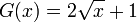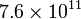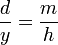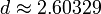## Thursday, 23 June 2011

### Non-Vedic Maths: Half and five for the odds

In my series of Non-Vedic maths I will present mental arithmetic techniques that allow you to speed up your number-crunching. In contrast to Vedic maths, I will also explain why these techniques work. I believe that being able to add and multiply quickly does not make a good mathematician. But understanding how things work and being able to develop your own techniques is more important.

Today's technique teaches how to multiply any number by 5, 50, 500 etc. The technique is based on the simple fact that

5 = 10/2


In this way multiplication by 5 is the same as multiplication by 10 and the division by 2. By using a technique for quickly dividing by 2 the method is accelerated further.

3465 × 5 = 34650 / 2


Dividing by two can be done in two ways. If you know your multiples of two up to 9×2=18 by heart then the traditional method is probably best. Just divide every digit from left to right including the carry over from the remainder. Note that the carry over is either 0 or 1, so the largest number that you will ever have to divide is 19. In our example we get

34650 / 2 = 17325


This is our result!

Some people are not as comfortable or quick with dividing numbers above 10 by 2. For these people the following alternative method might be faster. First divide every digit by 2 and forget about the remainder or carry over,

34650
12320


Next we shift the number to divide one place to the right (this was our original number) and replace every even digit with 0 and every odd digit with 5.

3465
5005


This second step effectively accounts for the carry overs that we missed in the first step. Now add the results of these two operations together

12320
5005
-----
17325


Because all the digits of the first number result from division of numbers less than ten, none of the digits can be larger than 4. For this reason we never have to worry about carry-overs when adding the two numbers.

For a slightly more mathematical explanation to what we have done here, let's write 34650/2 in the following way

34650/2 = (24640 + 10010)/2
= 24640/2 + 10010/2
= 12320 + 5005
= 17325


To summarise, in order to multiply a number by five

• append a zero at the right of the number
• divide every digit by two, ignoring any remainders
• add five to every digit to the right of an odd digit of the number that you got after the first step.

## Wednesday, 15 June 2011

### Catalan's theorem and Pillai's conjecture

Number theory always surprises by the seemingly simple questions which turn out to be extremely hard or maybe even impossible to answer. One such question concerns perfect powers of the type
xn
where both x and n are natural numbers. Can we say, which numbers can be expressed as perfect powers? If we don't further restrict n then the answer is simple. Any number x can be represented as x1. So that means the set of numbers that can be represented as perfect powers is the complete set$\mathbb{N}$. So this question is boring.

But what if we exclude these trivial powers and say that n must be greater than 1? The list of these numbers is the sequence A001597 in OEIS. On the right is a plot of the first 100 of these numbers an. As you can see, the numbers seem to grow more than linearly. In fact for large numbers they seem to grow with n2. This seems to suggest that the gaps between two consecutive numbers also needs to grow. This is intuitively right. As the numbers grow, there are less and less candidates that could produce perfect powets.

But how large are the gaps exactly? More interestingly, as the gaps grow larger, do we still encounter some small gaps? The plot on the left shows the differences gn between two consecutive elements of the sequence plotted against the smaller number for the first 1000 or so numbers
gn = an + 1an,
i.e. we're plotting gn against an. It can be seen that most of the gn seem to lie close to a curve given by$G(x)= 2 \sqrt{x} + 1$
which also limits the gn. This simple form is amazing at first, however it becomes quite clear when one looks at just the square numbers x2. If an is a square number then$(\sqrt{a_n}+1)^2$ is also a perfect square. The difference between the two numbers is$2 \sqrt{a_n}+1$. Only few numbers seem to depart from this behaviour and have a lot smaller values. This always happens when there is at least perfect power, that is not a square, between two perfect squares.
So we have an upper bound for the gn. But is there a lower bound for the gn that increases with n. Or does gn always have excursions to small numbers? Let's look first at the smallest possible value gn = 1.
Catalan's theorem makes a statement about this particular case. It says that there is only one n for which gn = 1, or in the full notation
xnym = 1 has only one solution, which is 32 − 23 = 1
Until 2002 this was Catalan's conjecture but then it was proven by Preda Mihăilescu. Long before this proof Pillai generalised the the Catalan conjecture and speculated about the solutions of the equation
xnym = c for c > 1
The graph above seems to suggest that for c = 2 there is also just one solution
33 − 52 = 2
but to my knowledge this has not been proven. Pillai's conjecture now states that for any value of c, the above equation only has a finite number of solutions. This means that the gaps in the sequence of perfect powers not only grow, but there is some increasing lower bound for the gaps as well. Erdos went one step further and conjectured that this lower bound is given by
gn < nk
where k is a fixed constant which is greater than one.
From the plot of the first million gaps this does not immediately seem obvious. The figure shows the plot of the gaps gn against n for n up to 106 on a double-logarithmic scale. Although the lower bound does seem to grow, the value of the parameter k of the Erdos formula, of roughly 0.22 (green line), is determined by a single outlier at a384026 = 143384152921 and g384026 = 17. Almaost all other values of gn lie well above the Erdos curve. Only one other point comes close to the limiting curve at g745174 = 24
One more interesting feature that can be seen are strange lines in the plot that slope at a slightly lower exponent of about 2 / 3. This suggests that there is some hidden structure in the seemingly random behaviour of the gn. Another feature, which I noticed when plotting the gn is that there seem to be islands of regularity.
If we zoom in to the region from$7.4\times10^{11}$ to$7.6\times 10^{11}$ we get the following plot. Most points are again crowded around the upper limit but the others have the very regular shape of two parabolas intersecting each other.
As you can see, even formulas that seem simple can have surprisingly rich behaviour and open up very difficult problems.

## Saturday, 11 June 2011

I have been following the development of the internet currency bitcoin for the last few days. I heard a lot of people talking about it, so I thought it might be an interesting option for an online currency. The value of a bitcoin has been steadily increasing over the last few weeks up to a record high of over 30$. Yesterday saw a development in the value of a bitcoin that made the media speak of a "Black Friday". I won't go into the discussion about the morality of this online currency as there have been reports that the drug trade is turning to bitcoins as a payment. But here I want to ask the question: Was the "Black Friday" really a crash? From the data I got from the mtgox.com API, I plotted the graph above. Naturally, we should plot any kind of exponential growth on a semi-logarithmic scale. The origin is some arbitrary point in the past, roughly 1000 hours before the time of writing this post. Older data is averaged over larger intervals, because it is not available any more as real-time data. One can see that, on average, the value of the currency has doubled every 7 days. I have also plotted an upper resistance and a lower support that both grow at this constant average growth rate. You can see that the value of a bitcoin has kept to this channel between its resistance and its support for almost 1000 hours. And even today it only made a very short excursion below the support curve. Note that it could have made this kind of excursion 200 hours ago as well. The averaged nature of the data for that time period makes it impossible to judge exactly what happened then. To be speaking of a "Black Friday" is therefore very premature. All that happened was that the over-exponential growth of the last few days has been compensated for. Of course it has to be seen wether the support will hold. But even if it doesn't, there is a good chance of a sideways trend to follow the rapid growth of the currency. My conscience dictates that I write a warning and disclaimer at the end of this post. I am not endorsing the bitcoin currency and I am not encouraging to invest or to trade in bitcoins. Please be very careful as to where you invest your money and don't take any online article as the ultimate truth about the matter. ## Thursday, 2 June 2011 ### Binary Trees in SQL: The Adjacency List When learning to program, a key skill is to know you data structures. For every task there is the ideal data structure that makes the task easy and straightforward to implement. But then we are thrown into the real world. In this real world we are told to finish a piece of code with the restriction that the data has to be stored in a SQL database. Most of the time we think of databases essentially being arrays in which the data is stored in a tabular way. In the back of our head we know that a more sophisticated data structure would be more fit for the task, but we don't have the time or can't be bothered to think about these luxuries in more detail. One prime example is the tree data structure. Wouldn't it be great if there was a simple, straightforward way to implement trees in SQL? I am going to show how this can be done. As it turns out there is more than one solution to the problem. In this post I will be writing about the first and maybe the most intuitive approach, the adjacency list. In the end this approach will turn out to be OK but not ideal for all applications. In two following posts I will then present more stable approaches to the problem, the nested set and the binary heap. ### Adjancency List The adjacency list is probably the implementation of a binary tree that is the easiest to understand and the one that most people would come up with if asked to implement a tree structure. Every entry in the tree connects two elements by a parent child relationship. We want to keep things neat and tidy, so we make sure that we separate data from structure. What this means is that we have a table with all the data, somewhat like this CREATE TABLE people (id INTEGER PRIMARY KEY NOT NULL, name VARCHAR(30));  Now we define the table that will hold the structure of the tree. Every entry in this tree will link a child node to a parent node and therefore has two references to our data table CREATE TABLE bintree (parent INTEGER, child INTEGER NOT NULL, rel CHAR(1));  You will notice an extra column 'rel' in this table which can hold the two values 'L' or 'R'. Because we are storing a binary tree, each node is either the left or the right node of it's parent. By specifying the relation to it's parent we can perform proper binary searches in the table. Keeping the two tables separate allows us to create the tree structure for the data relatively independently of the data itself. We could also sort the same data set in two or more trees for different sorting criteria. Now let's start populating our tables with data. When we insert a piece of data into our data table, we must also insert the relationship to the other data sets into the tree table. The next two insert statements do the job. INSERT INTO people VALUES (1,'John'),(2,'Ben'),(3,'Sue'); INSERT INTO bintree VALUES (-1,1,''),(1,2,'L'),(1,3,'R');  But note how we have to take care that we retain the tree structure. The parent must exist and it can have a maximum of one left and one right child node. Only the root node does not have a parent which is denoted by a -1 in the parent column. This constraint can be tested using check statements, but I will not go into the details of that in this post. Deleting a leaf node from the tree is also done using two simple drop statements, for example to remove Ben from the tree we execute the following DELETE FROM people WHERE id=2; DELETE FROM bintree WHERE child=2;  We have to ensure manually that the node deleted in this way doesn't have any children of its own. If it does, the children will end up orphaned and unreachable. Checking this can again be done using check statements. The real problem arises when you try to delete a node and all it's children. The only way to achieve this is by going through all the elements which are reachable from the node and delete them individually. This can be quite time consuming if you have a larger database. Another common task is to find out if one node A is the ancestor of another node C. Again, a number of SQL statements have to be issued. Starting from the child node C, we find it's parent SELECT parent FROM bintree WHERE child=[C]  If this returns the ancestor A then we know that the two nodes are related by an ancestor-descendant relationship. If the statement returns nothing, then we have reached the root of the tree telling us that the two nodes are not related. If we get any other node then we issue the same command again, but with the new node as the child node. We continue this way until we have either found the ancestor or reached the root node. The PHP code for this algorithm is as follows // a function that returns true if$parent is an ancestor of child and false otherwise
function isAncestor($parent,$child) {
$node =$child;
while ($node != -1) {$query="SELECT parent FROM bintree where child=".$node;$result=mysql_query($query); // should return exactly one result$node=mysql_result($result,0,"parent"); if ($node==\$parent) return true;
}
return false;
}


As you can see, the adjacency list layout for storing trees is not the ideal method. Some simple operations take multiple SQL queries and the consistency of the tree is not automatically ensured. Apart from orphaned nodes we have to avoid cyclic dependencies, where one node ends up to be it's own ancestor. In another post I will write about alternative methods that will alleviate some of these problems.

## Wednesday, 1 June 2011

Previously I posted the Two Ladders Puzzle in which two ladders lean against opposite walls. The length of the ladders is given and so is the height of the point where they intersect. The task was to find the distance between the walls. The problem can be solved using Pythagoras and the law of similar triangles, so really it can classify as an easy problem. Nonetheless, the answer requires the solution of a quartic equation. We begin by naming the lengths of the ladders a and b and the, as yet unknown heights at which the ladders lean against the walls by x and y. Also let's divide the distance between the two walls into the parts m and n. We now have 5 unknowns, so we need 5 equations. The easy one is

m + n = d

Then we have Pythagoras for the two ladders

a2 = d2 + x2

b2 = d2 + y2

Finally we have the similar triangles$\frac{d}{x} = \frac{n}{h}$$\frac{d}{y} = \frac{m}{h}$

If we add these two equations, we get the sum (m + n) / h on the right hand side. But m + n = d and thus we can eliminate d to obtain the nice intermediate result$\frac{1}{h} = \frac{1}{x} + \frac{1}{y}$

This means that h is the harmonic mean of x and y, independent of the values of a,b or d.

The rest of the solution will become a tiny bit messy. Instead of looking for a solution of d, we can look first for the values of x or y. Then we can use Pythagoras to find d. To do this we subtract the two Pythagorean equations to eliminate d

x2y2 = a2b2

Solving the harmonic equation for y we get$y = \frac{hx}{x-h}$

With this we can eliminate y from the above difference of squares$x^2-\frac{h^2 x^2}{(x-h)^2} = a^2-b^2$

Obviously this is a fourth order equation for x. I know this is not really a nice result so we will have to get some help for solving it. So let's insert the values and stick it into WolframAlpha. Of course there are four possible solutions. We get one positive, one negative and two complex solutions. Only the positive solution of$x \approx 3.03692$ is relevant. Now we can use the first Pythagoras to find the value for d$d \approx 2.60329$

## Friday, 27 May 2011

### Tutorial: Midpoint rule

In a previous post I spoke about the trapezium rule for integrating functions numerically. The trapezium rule could find the value of an integral with second order accuracy. In this post I will present an alternative method, the midpoint rule. The geometric interpretation of the midpoint rule appears to be more crude than the trapezium rule. Nonetheless, it turns out that the midpoint rule also has second order accuracy.

Like the trapezium rule, we divide our interval [a;b] into N equal sections of width h = (ba) / N. The area of each section is now approximated by the area of the rectangle of width h and whose height is given by the function value at the centre of the section. The centre of the nth section is given by
xn + 1 / 2 = a + (n + 1 / 2)h.

Here n = 0,1,...,N − 1. As before, we define fn + 1 / 2 = f(xn + 1 / 2). The area of the nth rectangle is then given by hfn + 1 / 2. And the value of the integral is given by$\int_a^b f(x)\;dx = h( f_{1/2} + f_{3/2} + \ldots + f_{N-1/2}) = h\sum_{k=0}^{N-1}f_{k + 1/2}$

This is the final form for the midpoint rule. As with the trapezium rule, the accuracy of the integral can be increased by increasing N, i.e. decreasing the step size h. Again we can plot the error of the numerical integral against the number of steps on a logarithmic scale.

In figure the error of the integral$\int_0^1\sin(\pi x) dx = \frac{2}{\pi}$

when evaluated with the midpoint and the trapezium rule is plotted. Clearly, the midpoint rule has a second order error, as has the trapezium rule. At first sight this is somewhat surprising since the midpoint rule makes no attempt to incorporate the change in the function value over the sections into the approximation.

To understand the accuracy of the approximation, we have to re-interpret the midpoint formula hfn + 1 / 2 as the area of a trapezoid that intersects the function graph at the midpoint an which has a slope identical to the derivative of the function at that point. It is a simple, but very useful, geometric fact that the area of a trapezoid is the same as the area of a rectangle whose height is the average of the trapezoid's height.

Looking at the error curves a bit more closely, we can observe that the error of the midpoint rule is always slightly smaller than that of the trapezium rule. In fact when we divide the two error curves in the region where they decrease with N^2, we get an almost constant error ratio of about 1/2. This will lead us to improve the two methods. But this will be the subject of another post.